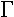# Mismatch Loss, Etc.

On this page we will discuss mismatch loss, as well as "loss factor" (as described in a recent trade journal article), and a new concept, "efficiency factor".

### Mismatch loss

Mismatch loss is the ratio of power delivered, to power available (thanks for the correction, David!) The formula for mismatch loss is simply:

mismatch loss=(1-2)

We've done the heavy lifting for you, and already put it into a calculator, check it out!

### Loss factor

This concept is described in recent a Microwave Journal article entitled "Automation and Real-time Verification of Passive Component S-parameter Measurements Using Loss Factor Calculations", by J. Capwell, T. Weller, D. Markell and L. Dunleavy of Modelithics Inc. You can google to it if you like, but to get the full text you might have to join the Microwave Journal web site, which is annoying. You can always read the google "cached" version of the article, that's what we did.

They present the concept of "loss factor" as something that will help you determine if an S-parameter measurement of a passive device is good. Quoting the article:

"The forward and reverse loss factors are calculated from passive component S-parameters as

Forward Loss Factor (FLF) = 1 - |S11|2 - |S21|2

Reverse Loss Factor (RLF) = 1 - |S22|2 - |S12|2

By these definitions, the loss factors are seen to equal the difference between a normalized input power and the power that is reflected and transmitted to the input and output ports, respectively. (Power loss can occur due to conductor, dielectric and radiation loss mechanisms.) For reciprocal devices S21 = S12, the differences between the forward and reverse loss factor occur due to differences in |S11| and |S22|. For ideal lossless components, the magnitudes of S11 and S22 are equal, but they can deviate from one another whenever loss is present.

For passive components such as capacitors, inductors and resistors (and diodes), the electrical behavior is most often symmetrical (S11 = S22); thus, the difference in the forward and reverse loss should be negligible. Significant deviations in the forward and reverse loss can be observed when a component begins to radiate, a commonly observed phenomenon, particularly for inductors. However, the main objective of this article is to illustrate how real-time monitoring of loss factor behavior becomes a useful tool for detecting measurement inconsistencies when, all things functioning properly, the component should exhibit symmetrical characteristics."

We agree that loss factor is a one indication of how much power is "disappearing" in a network, through resistive loss or radiation.

### Efficiency factor

This a Microwaves101 concept, which we believe is a more useful quantity than "loss factor" for evaluating passive parts. We define it as:

Forward efficiency factor = |S11|2 +|S21|2

Reverse efficiency factor = |S22|2 +|S12|2

Why is this better? It all makes sense when converted to decibels. A "perfect" circuit has an efficiency factor of 0 dB. A circuit that loses 20 percent of its power has an efficiency factor of -1 dB, etc. etc. Now you have a measurement of how "lossless" a circuit would be if you were able to perfectly impedance match it. This is quite useful when you are designing low-loss networks such as switches. Of course, Microwaves101 ain't making the big bucks like Modelithics is, so you have to factor that in when you read this page...

Check out our S-parameter Utility spreadsheet page, it has an example that where mismatch loss, loss factor and efficiency factor are plotted and analyzed.

Author : Unknown Editor